##### Chemistry II For Dummies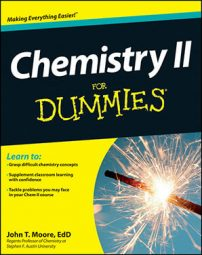Chemistry II is more than fires and smelly explosions. Chemistry II is more about solving calculations. In fact, Chemistry II has a lot more calculations and math than your Chemistry I class did. In your Chemistry II class, you need to master several formulas so you can calculate different mathematical problems, ranging from kinetics, different types of equilibrium, thermochemistry, and electrochemistry.

This Cheat Sheet can serve as a quick reference to how to solve kinetics, thermodynamics, and different types of equilibrium problems.

## How to calculate kinetics problems

The study of kinetics, the speed of chemical reactions, is essential to the study of chemistry and is a major topic in any Chemistry II class. Knowing the concepts of kinetics can help your understanding of why some reactions are fast and others slow and why some simple reactions are slow and other, more complex reactions are fast.

The reaction rate (the speed of reaction) is the change in the concentration of a reactant or product per the change in time. You can write it as: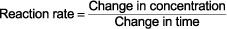Chemists normally measure concentration in terms of molarity, M, and time is usually expressed in seconds, s, which means that the units of the reaction rate are M/s. You can express the number of units in other ways such as: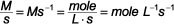## How to solve homogeneous equilibrium problems

The equilibrium constant describes the relationship between the amounts of the reactants and the products at a certain temperature. You’ll need to know the equilibrium constant as you study Chemistry II. For the general equilibrium: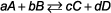the equilibrium constant expression is: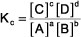In the expression, K is the equilibrium constant, the subscript c indicates this constant is expressed in terms of concentrations (not pressures, p), the brackets (as usual) stand for molar (moles/L) concentration, the uppercase letters are the reactant and product species, and the lowercase superscripts are the coefficients in the balanced chemical equation.

## Solving acid-base equilibrium problems

The acid and base equilibrium constant expressions describe the relationship between the amounts of reactants and products in aqueous acid-base systems. For the following general weak-acid equilibrium: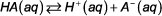the equilibrium constant expression is: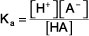For a general weak-base equilibrium: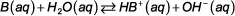the equilibrium constant expression is:The concentration of water (or any pure liquid or solvent or solid) does appear in the equilibrium constant expression. K is the equilibrium constant, the subscript b indicates that this is an equilibrium constant expression for a weak base, and the brackets indicate molar concentrations.

## Calculating solubility equilibrium problems

The solubility product equation is used to describe the equilibrium situation when a not-so-soluble salt is dissolving in water. For the general dissociation of a sparingly soluble salt: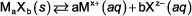In this equation, x+ and z– are the magnitude of the positive and negative charge, respectively; the equilibrium constant expression (solubility product expression) is

Ksp = [Mx+]a[Xz]b

## Tackling thermodynamics problems

The Gibbs Free Energy is the best indicator about whether a reaction will be spontaneous or nonspontaneous. You’ll need to know this as you study Chemistry II. It has the form: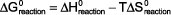In this equation ΔG° is the Gibbs Free Energy of a reaction under standard conditions of 1 atm (or 1 bar) for gases and 1 M for solutions at 25°C; ΔH° is the enthalpy of the reaction under standard conditions; T is the Kelvin temperature; and ΔS° is the entropy of the reaction under standard conditions.

A spontaneous process has °G < 0. A nonspontaneous process has ΔG > 0. When °G = 0, the process is at equilibrium.